# 利用预训练语言模型拓展实体集合

🎎 Reading Group 的 pdf 版本

Empower Entity Set Expansion via Language Model Probing. ACL 2020.
Yunyi Zhang, Jiaming Shen, Jingbo Shang, Jiawei Han

1. 单纯的相似度计算，容易拓展不准确的实体(非同级，反义词，High level 相似，但细粒度不同).
2. 随着集合的拓展，容易出现语义偏移等误差累计问题.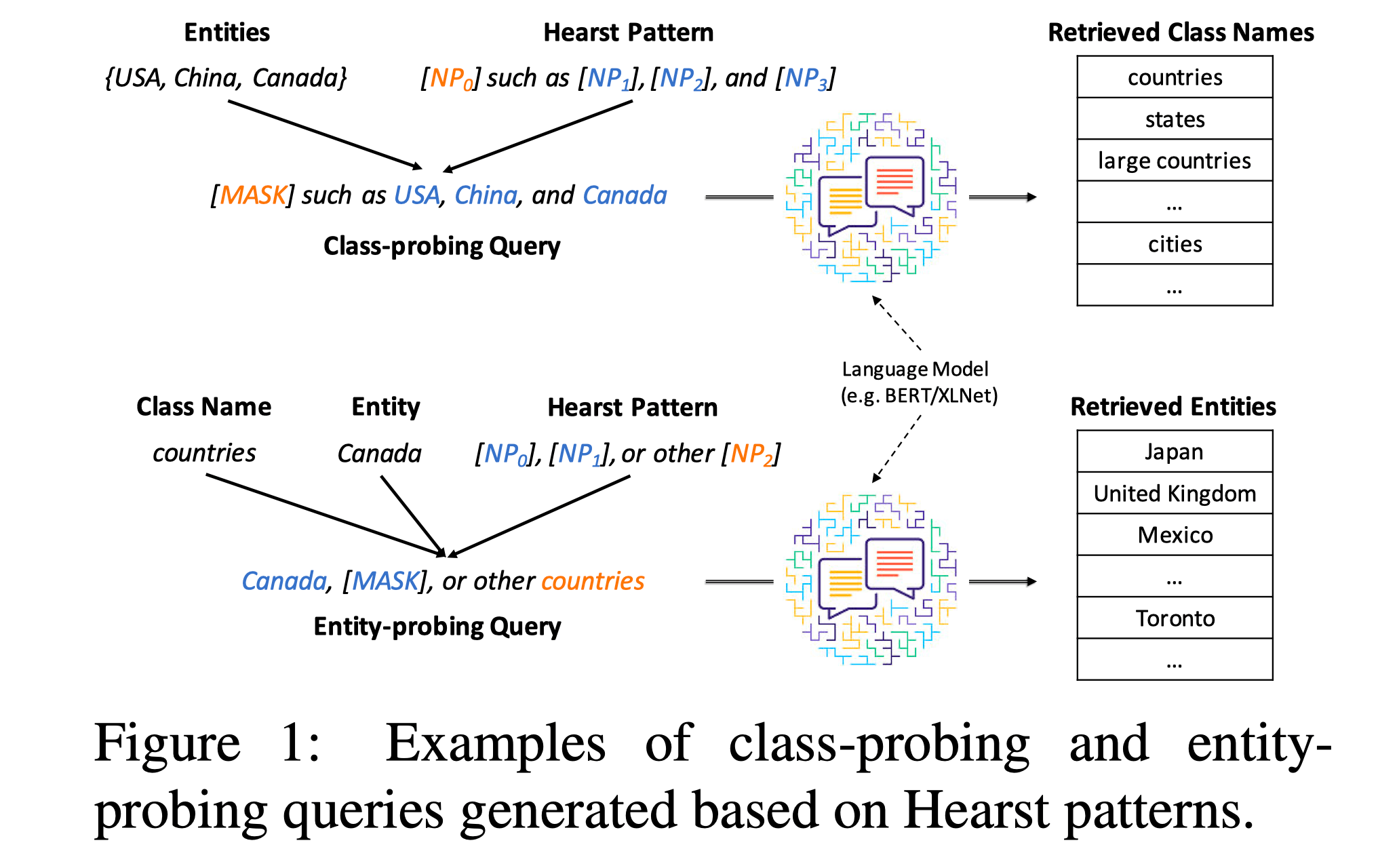## Class-guided Entity Expansion

• 集合类名生成(class name generation).
• 集合名称排序(class name ranking).
• 集合指导下的实体选择(class-guided entity selection).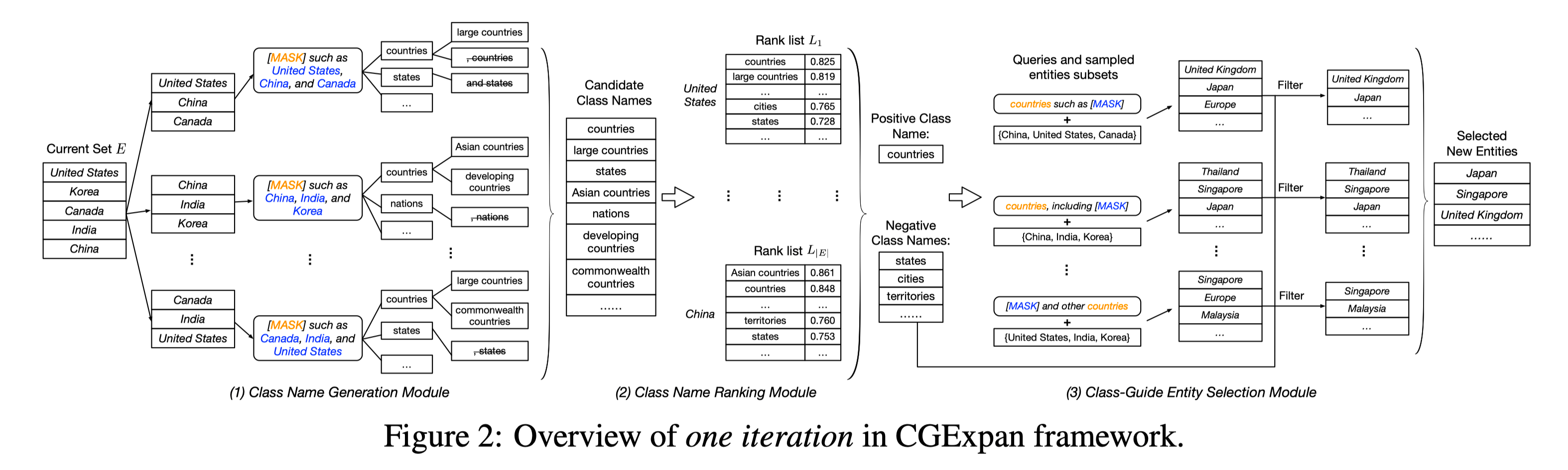### Class Name Generation

- NPy such as NPa
- such NPy as NPa
- NPa or other NPy
- NPa and other NPy
- NPy, including NPa
- NPy, especially NPa


### Class Name Ranking

M k ( e , c ) = 1 k max ⁡ X X e , ∣ X ∣ = k ∑ x ∈ X max ⁡ x ′ ∈ X c cos ⁡ ( x , x ′ ) M^{k}(e, c)=\frac{1}{k} \max_{X X_{e},|X|=k} \sum_{{x} \in X} \max_{{x}^{\prime} \in X_{c}} \cos \left({x}, {x}^{\prime}\right)

X e X_e 表示语料集中所有 entity 的 representation， X c X_c 表示 Hearst pattern 用 MASK 遮掉实体词的 representation.

### Class-guided Entity Selection

• Local score, s c o r e i l o c = M k ( e i , c p ) {score}_{i}^{l o c}=M^{k}\left(e_{i}, c_{p}\right)
• Global score, s c o r e i g l b = 1 ∣ E s ∣ ∑ e ∈ E s cos ⁡ ( v e i , v e ) {score}_{i}^{g l b}=\frac{1}{\left|E_{s}\right|} \sum_{e \in E_{s}} \cos \left({v}_{e_{i}}, {v}_{e}\right)

1. 排名的倒数. s t ( e i ) = 1 r i t s^{t}\left(e_{i}\right)=\frac{1}{r_{i}^{t}}
2. 相对 score. s t ( e i ) = s c o r e i t − min ⁡ e j ∈ R t s c o r e j t max ⁡ e j ∈ R t s c o r e j t − min ⁡ e j ∈ R t s c o r e j t s^{t}\left(e_{i}\right)=\frac{{score}_{i}^{t}-\min_{e_{j} \in R^{t}} {score}_{j}^{t}}{\max_{e_{j} \in R^{t}} {score}_{j}^{t}-\min_{e_{j} \in R^{t}} {score}_{j}^{t}}

## Experiments

Wiki 是 Wikipedia Corpus 的子集，APR 则是 15 年的一个新闻数据集。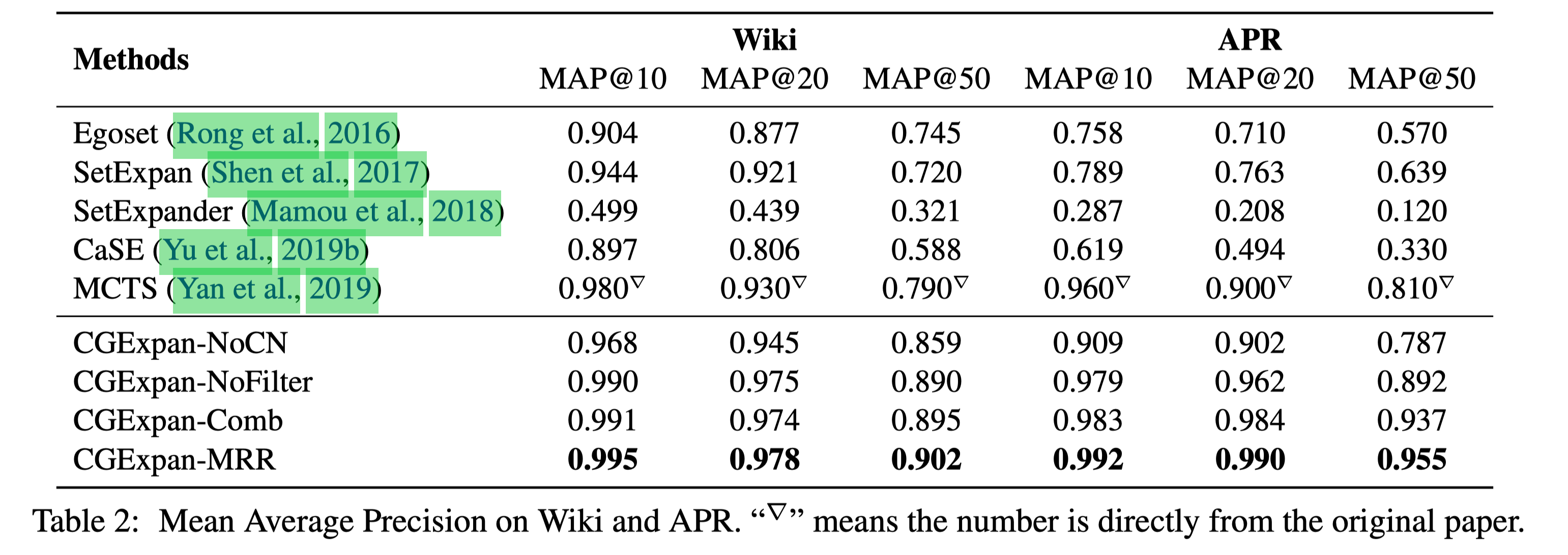Ablation experiments 中，NoCN 是去掉 Class name 模块直接计算 score，相对于一个只用 BERT representation 的方案。

NoFilter 在 Wiki 上差异不大，在 APR 上就比较大了，可能是 BERT 已经能很好控制 Wikipedia 语料中语义的情况。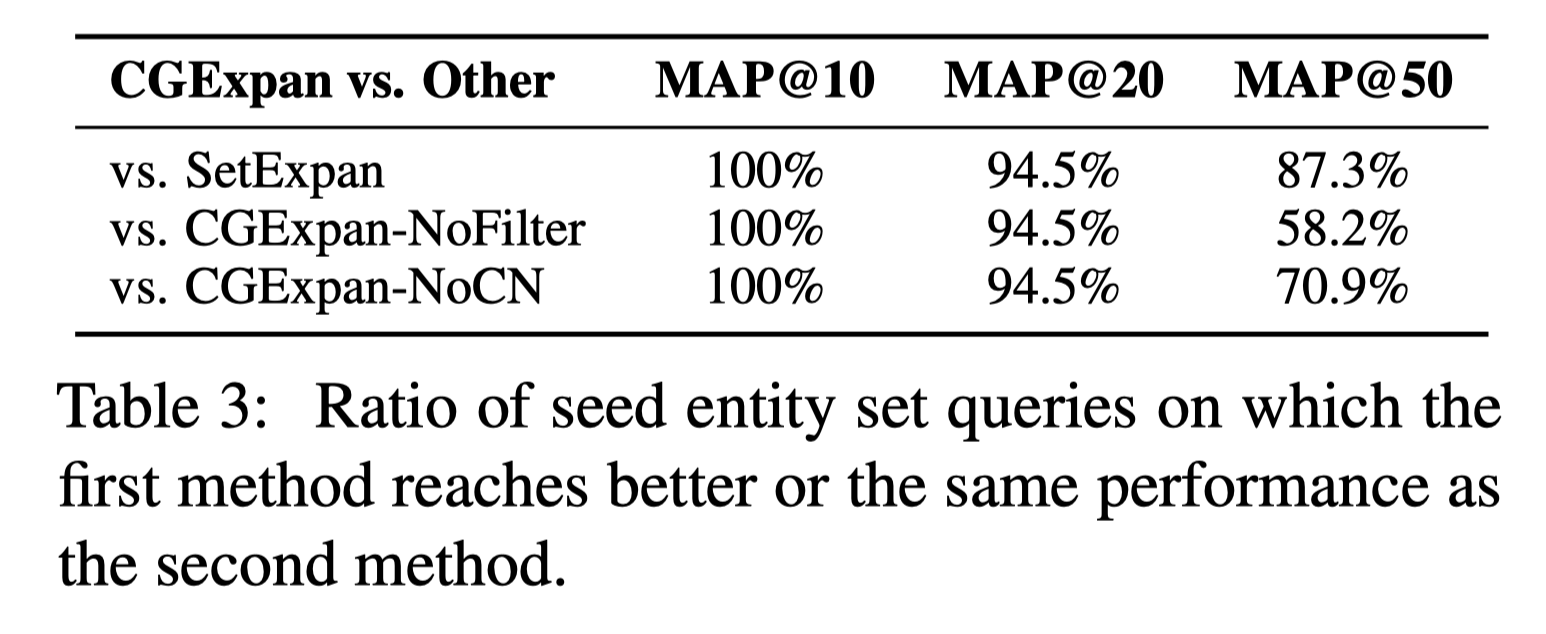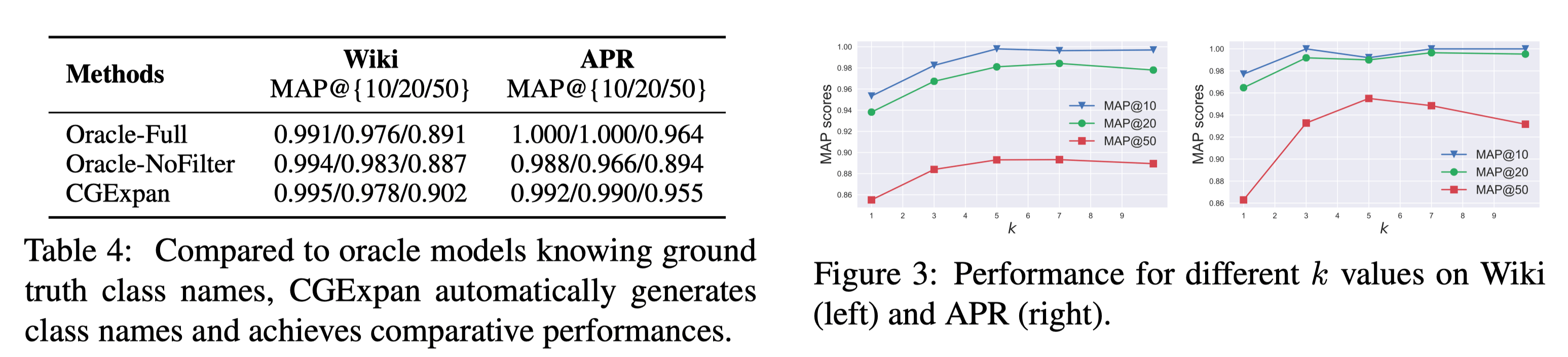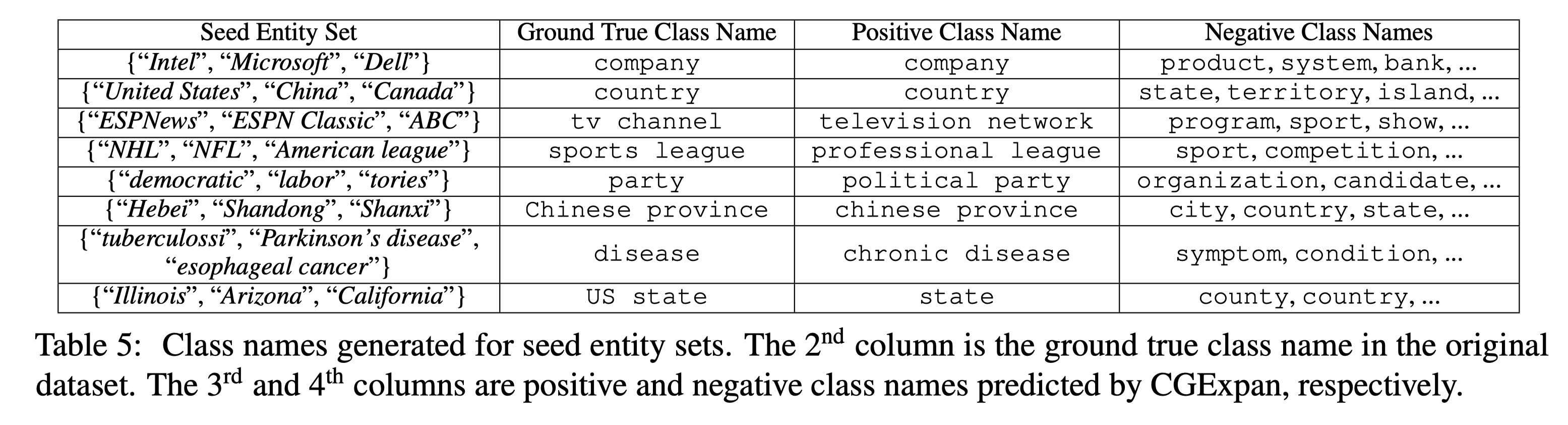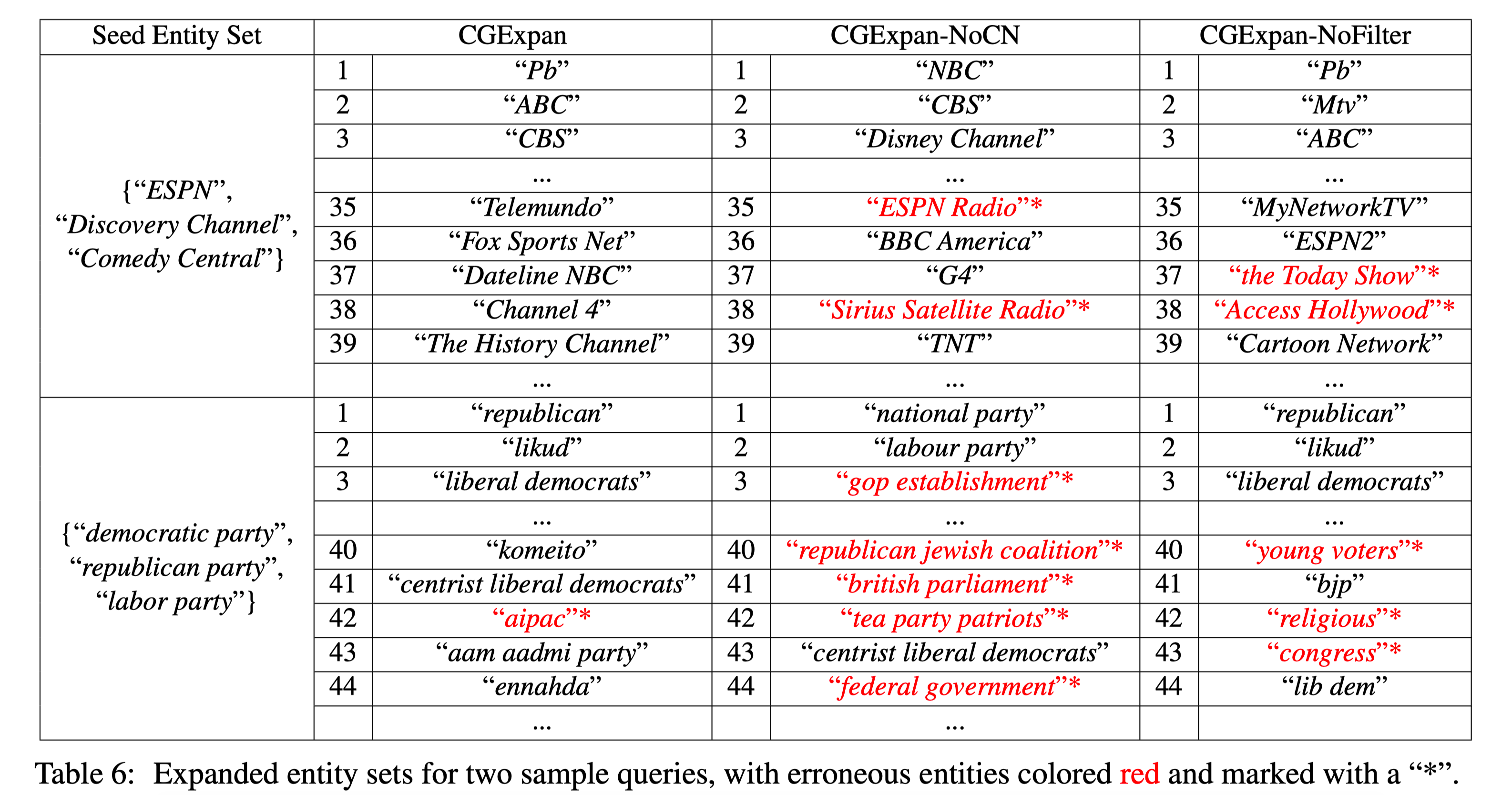Case study 中可以看见 negative class name 很高质量，能够分离特别相近的语义。

## Discuss

1. CGExpan 是一种从预训练 LMs 中提取信息的一种模式。
2. 不需要 train，也使得 pipeline 的误差传递会比较少。
3. class name, filter 策略效果还是比较明显的。
4. 但目前 entity set 只有 55 个，样本量整体偏小，另外 wiki 被 BERT 训过，泛化性需要进一步考证。
5. 不过这套模式，扩展性比较强，可以用于主题扩展，商品扩展等。作者也写了一下 future work，比如说扩展抽象概念，构建语义族。
6. 如果有 pair-wise 的对比实验就更好了

11-094484
04-11109602-23754
03-10695
10-101567
06-25300
08-231446
11-17402
04-13830
01-29892